# The Metabiaugmented Dodecahedron

The metabiaugmented dodecahedron (J60) is the 60th Johnson solid. It has 22 vertices, 40 edges, and 20 faces (10 pentagons and 10 triangles). It can be constructed augmenting a dodecahedron with two pentagonal pyramids separated by an edge.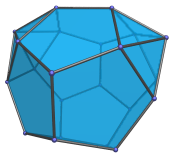If one of the pentagonal pyramids is removed, the result is the augmented dodecahedron (J58). If instead a third pyramid is added to a face non-adjacent to either augment, the triaugmented dodecahedron (J61) is produced.

If the pentagonal pyramids are erected over opposite pentagons instead, the result is the parabiaugmented dodecahedron (J59).

## Projections

Here are some views of the metabiaugmented dodecahedron from various angles:

Projection Description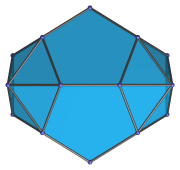Top view.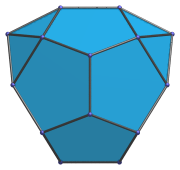Front view.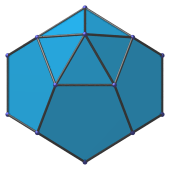Side view.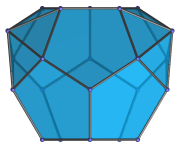Parallel to pair of opposite pentagons.

## Coordinates

The Cartesian coordinates of the metabiaugmented dodecahedron, centered on the origin and with edge length 2, are:

• (0, ±φ2, ±1)
• (±1, 0, ±φ2)
• (±φ2, ±1, 0)
• (±φ, ±φ, ±φ)
• (0, (7+φ)/5, ±(8φ+1)/5)

where φ=(1+√5)/2 is the Golden Ratio.

Last updated 16 Jan 2019.